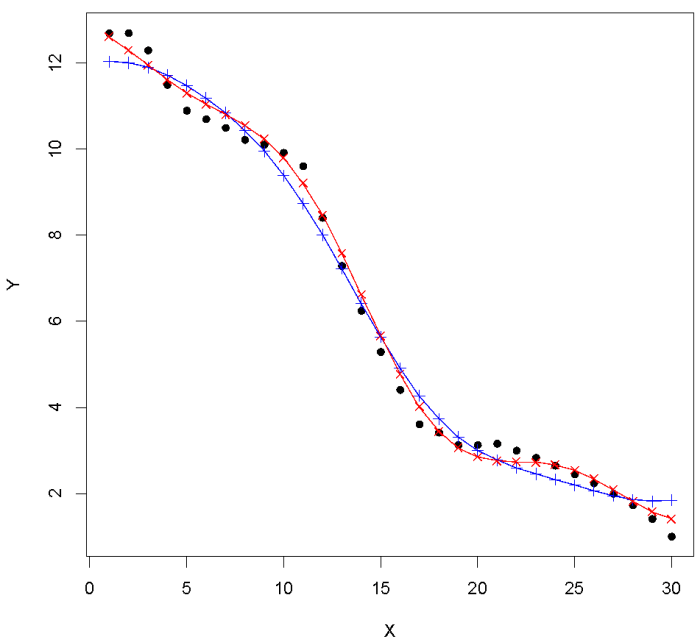Select Page

## Optimization Using R

Optimization is a technique for finding out the best possible solution for a given problem for all the possible solutions. Optimization uses a rigorous mathematical model to find out the most efficient solution to the given problem. What is optimization? Optimization...## Building Regression Models in R using Support Vector Regression

The article studies the advantage of Support Vector Regression (SVR) over Simple Linear Regression (SLR) models for predicting real values, using the same basic idea as Support Vector Machines (SVM) use for classification. The article studies the advantage of Support...## Tips for Getting Started with Text Mining in R and Python

It All Starts With The Text There is so much of information lying in the text posts made by you and me and all others about all the trending topics today. Being in our respective firms, big or small, each of us collect some data related to our respective businesses...
Page 4 of 21« First...23456...1020...Last »